Elementary Algebra 2e

# 4.3Graph with Intercepts

Elementary Algebra 2e4.3 Graph with Intercepts

### Learning Objectives

By the end of this section, you will be able to:

• Identify the x - and y - intercepts on a graph
• Find the x - and y - intercepts from an equation of a line
• Graph a line using the intercepts

### Be Prepared 4.6

Before you get started, take this readiness quiz.

Solve: $3·0+4y=−2.3·0+4y=−2.$
If you missed this problem, review Example 2.17.

### Identify the x- and y- Intercepts on a Graph

Every linear equation can be represented by a unique line that shows all the solutions of the equation. We have seen that when graphing a line by plotting points, you can use any three solutions to graph. This means that two people graphing the line might use different sets of three points.

At first glance, their two lines might not appear to be the same, since they would have different points labeled. But if all the work was done correctly, the lines should be exactly the same. One way to recognize that they are indeed the same line is to look at where the line crosses the x- axis and the y- axis. These points are called the intercepts of the line.

### Intercepts of a Line

The points where a line crosses the x- axis and the y- axis are called the intercepts of a line.

Let’s look at the graphs of the lines in Figure 4.18.

Figure 4.18 Examples of graphs crossing the x-negative axis.

First, notice where each of these lines crosses the $xx$ negative axis. See Figure 4.18.

 Figure The line crosses the x- axis at: Ordered pair of this point Figure (a) 3 $(3,0)(3,0)$ Figure (b) 4 $(4,0)(4,0)$ Figure (c) 5 $(5,0)(5,0)$ Figure (d) 0 $(0,0)(0,0)$
Table 4.24

Do you see a pattern?

For each row, the y- coordinate of the point where the line crosses the x- axis is zero. The point where the line crosses the x- axis has the form $(a,0)(a,0)$ and is called the x- intercept of a line. The x- intercept occurs when $yy$ is zero.

Now, let’s look at the points where these lines cross the y- axis. See Table 4.25.

 Figure The line crosses the y-axis at: Ordered pair for this point Figure (a) 6 $(0,6)(0,6)$ Figure (b) $−3−3$ $(0,−3)(0,−3)$ Figure (c) $−5−5$ $(0,−5)(0,−5)$ Figure (d) 0 $(0,0)(0,0)$
Table 4.25

What is the pattern here?

In each row, the x- coordinate of the point where the line crosses the y- axis is zero. The point where the line crosses the y- axis has the form $(0,b)(0,b)$ and is called the y- intercept of the line. The y- intercept occurs when $xx$ is zero.

### x- intercept and y- intercept of a line

The x- intercept is the point $(a,0)(a,0)$ where the line crosses the x- axis.

The y- intercept is the point $(0,b)(0,b)$ where the line crosses the y- axis.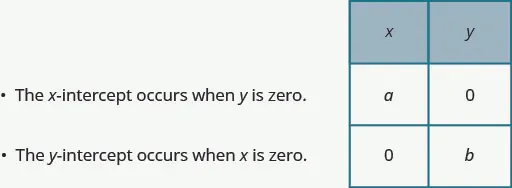### Example 4.19

Find the x- and y- intercepts on each graph.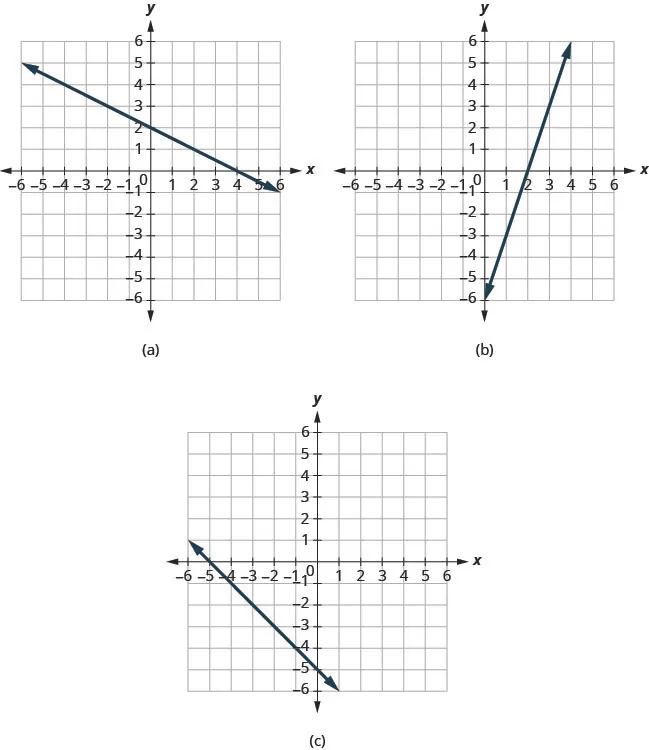### Try It 4.37

Find the x- and y- intercepts on the graph.### Try It 4.38

Find the x- and y- intercepts on the graph.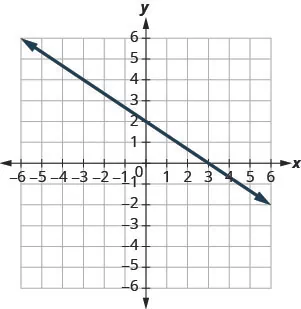### Find the x- and y- Intercepts from an Equation of a Line

Recognizing that the x- intercept occurs when y is zero and that the y- intercept occurs when x is zero, gives us a method to find the intercepts of a line from its equation. To find the x- intercept, let $y=0y=0$ and solve for x. To find the y- intercept, let $x=0x=0$ and solve for y.

### Find the x- and y- Intercepts from the Equation of a Line

Use the equation of the line. To find:

• the x- intercept of the line, let $y=0 y=0$ and solve for $xx$.
• the y- intercept of the line, let $x=0x=0$ and solve for $yy$.

### Example 4.20

Find the intercepts of $2x+y=62x+y=6$.

### Try It 4.39

Find the intercepts of $3x+y=12.3x+y=12.$

### Try It 4.40

Find the intercepts of $x+4y=8.x+4y=8.$

### Example 4.21

Find the intercepts of $4x–3y=124x–3y=12$.

### Try It 4.41

Find the intercepts of $3x–4y=12.3x–4y=12.$

### Try It 4.42

Find the intercepts of $2x–4y=8.2x–4y=8.$

### Graph a Line Using the Intercepts

To graph a linear equation by plotting points, you need to find three points whose coordinates are solutions to the equation. You can use the x- and y- intercepts as two of your three points. Find the intercepts, and then find a third point to ensure accuracy. Make sure the points line up—then draw the line. This method is often the quickest way to graph a line.

### Example 4.22

#### How to Graph a Line Using Intercepts

Graph $–x+2y=6–x+2y=6$ using the intercepts.

### Try It 4.43

Graph $x–2y=4x–2y=4$ using the intercepts.

### Try It 4.44

Graph $–x+3y=6–x+3y=6$ using the intercepts.

The steps to graph a linear equation using the intercepts are summarized below.

### How To

#### Graph a linear equation using the intercepts.

1. Step 1.
Find the x- and y- intercepts of the line.
• Let $y=0y=0$ and solve for $xx$
• Let $x=0x=0$ and solve for $yy$.
2. Step 2. Find a third solution to the equation.
3. Step 3. Plot the three points and check that they line up.
4. Step 4. Draw the line.

### Example 4.23

Graph $4x–3y=124x–3y=12$ using the intercepts.

### Try It 4.45

Graph $5x–2y=105x–2y=10$ using the intercepts.

### Try It 4.46

Graph $3x–4y=123x–4y=12$ using the intercepts.

### Example 4.24

Graph $y=5xy=5x$ using the intercepts.

### Try It 4.47

Graph $y=4xy=4x$ using the intercepts.

### Try It 4.48

Graph $y=−xy=−x$ the intercepts.

### Section 4.3 Exercises

#### Practice Makes Perfect

Identify the x- and y- Intercepts on a Graph

In the following exercises, find the x- and y- intercepts on each graph.

139.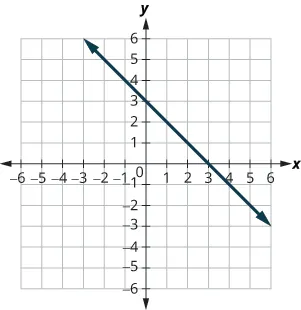140.141.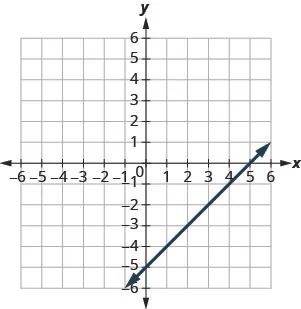142.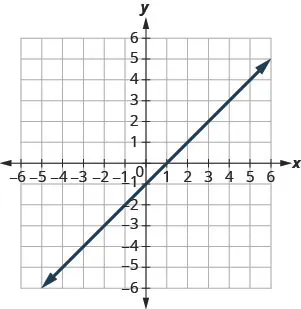143.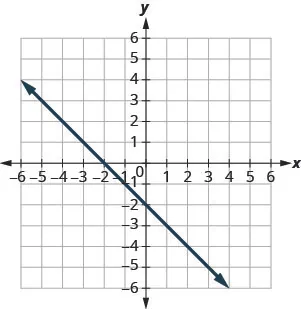144.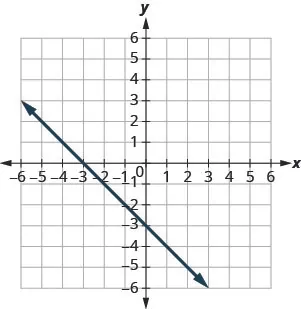145.146.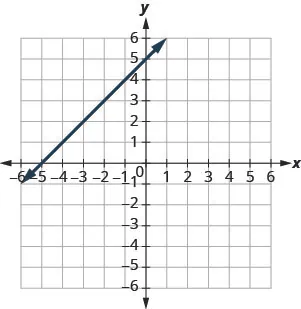147.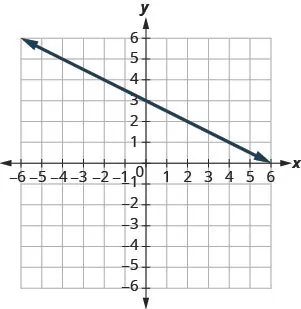148.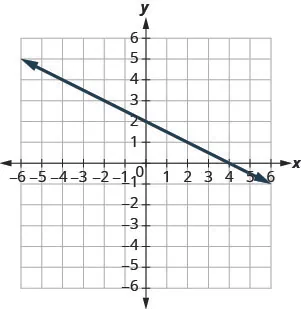149.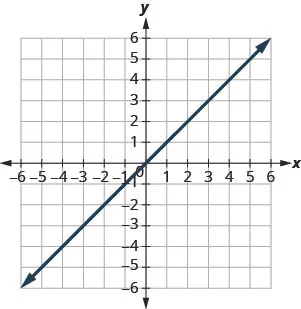150.Find the x- and y- Intercepts from an Equation of a Line

In the following exercises, find the intercepts for each equation.

151.

$x + y = 4 x + y = 4$

152.

$x + y = 3 x + y = 3$

153.

$x + y = −2 x + y = −2$

154.

$x + y = −5 x + y = −5$

155.

$x – y = 5 x – y = 5$

156.

$x – y = 1 x – y = 1$

157.

$x – y = −3 x – y = −3$

158.

$x – y = −4 x – y = −4$

159.

$x + 2 y = 8 x + 2 y = 8$

160.

$x + 2 y = 10 x + 2 y = 10$

161.

$3 x + y = 6 3 x + y = 6$

162.

$3 x + y = 9 3 x + y = 9$

163.

$x – 3 y = 12 x – 3 y = 12$

164.

$x – 2 y = 8 x – 2 y = 8$

165.

$4 x – y = 8 4 x – y = 8$

166.

$5 x – y = 5 5 x – y = 5$

167.

$2 x + 5 y = 10 2 x + 5 y = 10$

168.

$2 x + 3 y = 6 2 x + 3 y = 6$

169.

$3 x – 2 y = 12 3 x – 2 y = 12$

170.

$3 x – 5 y = 30 3 x – 5 y = 30$

171.

$y = 1 3 x + 1 y = 1 3 x + 1$

172.

$y = 1 4 x − 1 y = 1 4 x − 1$

173.

$y = 1 5 x + 2 y = 1 5 x + 2$

174.

$y = 1 3 x + 4 y = 1 3 x + 4$

175.

$y = 3 x y = 3 x$

176.

$y = −2 x y = −2 x$

177.

$y = −4 x y = −4 x$

178.

$y = 5 x y = 5 x$

Graph a Line Using the Intercepts

In the following exercises, graph using the intercepts.

179.

$– x + 5 y = 10 – x + 5 y = 10$

180.

$– x + 4 y = 8 – x + 4 y = 8$

181.

$x + 2 y = 4 x + 2 y = 4$

182.

$x + 2 y = 6 x + 2 y = 6$

183.

$x + y = 2 x + y = 2$

184.

$x + y = 5 x + y = 5$

185.

$x + y = −3 x + y = −3$

186.

$x + y = −1 x + y = −1$

187.

$x – y = 1 x – y = 1$

188.

$x – y = 2 x – y = 2$

189.

$x – y = −4 x – y = −4$

190.

$x – y = −3 x – y = −3$

191.

$4 x + y = 4 4 x + y = 4$

192.

$3 x + y = 3 3 x + y = 3$

193.

$2 x + 4 y = 12 2 x + 4 y = 12$

194.

$3 x + 2 y = 12 3 x + 2 y = 12$

195.

$3 x – 2 y = 6 3 x – 2 y = 6$

196.

$5 x – 2 y = 10 5 x – 2 y = 10$

197.

$2 x – 5 y = −20 2 x – 5 y = −20$

198.

$3 x – 4 y = −12 3 x – 4 y = −12$

199.

$3 x – y = −6 3 x – y = −6$

200.

$2 x – y = −8 2 x – y = −8$

201.

$y = −2 x y = −2 x$

202.

$y = −4 x y = −4 x$

203.

$y = x y = x$

204.

$y = 3 x y = 3 x$

#### Everyday Math

205.

Road trip. Damien is driving from Chicago to Denver, a distance of 1000 miles. The x- axis on the graph below shows the time in hours since Damien left Chicago. The y- axis represents the distance he has left to drive.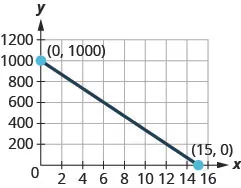1. Find the x- and y- intercepts.
2. Explain what the x- and y- intercepts mean for Damien.
206.

Road trip. Ozzie filled up the gas tank of his truck and headed out on a road trip. The x- axis on the graph below shows the number of miles Ozzie drove since filling up. The y- axis represents the number of gallons of gas in the truck’s gas tank.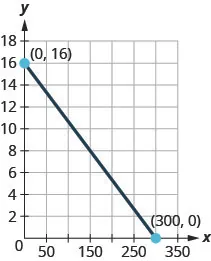1. Find the x- and y- intercepts.
2. Explain what the x- and y- intercepts mean for Ozzie.

#### Writing Exercises

207.

How do you find the x- intercept of the graph of $3x–2y=63x–2y=6$?

208.

Do you prefer to use the method of plotting points or the method using the intercepts to graph the equation $4x+y=−44x+y=−4$? Why?

209.

Do you prefer to use the method of plotting points or the method using the intercepts to graph the equation $y=23x−2y=23x−2$? Why?

210.

Do you prefer to use the method of plotting points or the method using the intercepts to graph the equation $y=6y=6$? Why?

#### Self Check

After completing the exercises, use this checklist to evaluate your mastery of the objectives of this section.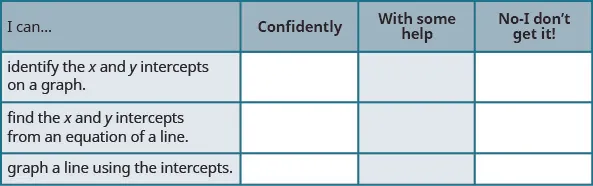What does this checklist tell you about your mastery of this section? What steps will you take to improve?

Order a print copy

As an Amazon Associate we earn from qualifying purchases.